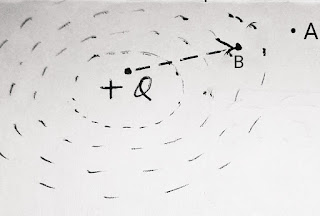# Electric Potential by A.K.Sinha

Concept of Electric Potential :

We know that a charge shows its effect in its vicinity known as electric field. If a test charge is put outside the vicinity or region of a source charge then the test charge does not experience any interaction due to the source charge. but if we try to put the test charge from outside of vicinity of a source charge to a point inside the vicinity then we have to do some work on the test charge.

Let us consider an electric field due to a  positive source charge. Now if we try to put a test positive charge in a point inside this electric field then we have to do some work against the field because the test charge will experience a repulsion due to source charge. This work done is saved in the test charge as its potential energy at that point.Fig : 1

We can define the Electric Potential of a point inside an electric field as the work done in bringing a unit positive charge from infinity to that point.

Hence, if a charge q is brought from infinity to a point in the electric field then electric potential at that point is given byWhere, V = Electric Potential

and  W = Work done in bringing that

charge from infinity to that

point in electric field

( Note : In electrostatic we use the term ' infinity '  to mention a position or space outside the electric field  or far away from source charge where it's effect can not be experienced. )

Electric potential is a Scalar quantity. But it describes the flow of charge between two charged body in contact. The charge will flow from a body at higher potential to a body at lower potential until the two body came in equal potential.

SI Unit :  V  or  volt.

Definition of 1 volt : Electric Potential at a point in electric field is called 1 volt or unit volt, If 1 joule of work is done in bringing a charge of 1 C from infinity to that point in the electric field.